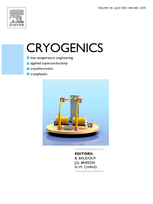Share

# The optimization on flow scheme of helium liquefier with genetic algorithm.

Author(s) : Wang H. R., XIONG L. Y., PENG N., et al.

Type of article: Article

Summary

There are several ways to organize the flow scheme of the helium liquefiers, such as arranging the expanders in parallel (reverse Brayton stage) or in series (modified Brayton stages). In this paper, the inlet mass flow and temperatures of expanders in Collins cycle are optimized using genetic algorithm (GA). Results show that maximum liquefaction rate can be obtained when the system is working at the optimal parameters. However, the reliability of the system is not well due to high wheel speed of the first turbine. Study
shows that the scheme in which expanders are arranged in series with heat exchangers between them has higher operation reliability but lower plant efficiency when working at the same situation. Considering both liquefaction rate and system stability, another flow scheme is put forward hoping to solve the dilemma. The three configurations are compared from different aspects, they are respectively economic cost, heat exchanger size, system reliability and exergy efficiency. In addition, the effect of heat capacity ratio on heat transfer efficiency is discussed. A conclusion of choosing liquefier configuration is given in the end, which is meaningful for the optimal design of helium liquefier.

Details